Next: Driven LCR Circuits Up: Damped and Driven Harmonic Previous: LCR Circuits

# Driven Damped Harmonic Oscillation

We saw earlier, in Section 3.1, that if a damped mechanical oscillator is set into motion then the oscillations eventually die away due to frictional energy losses. In fact, the only way of maintaining the amplitude of a damped oscillator is to continuously feed energy into the system in such a manner as to offset the frictional losses. A steady (i.e., constant amplitude) oscillation of this type is called driven damped harmonic oscillation. Consider a modified version of the mass-spring system investigated in Section 3.1 in which one end of the spring is attached to the mass, and the other to a moving piston. (See Figure 9.) Let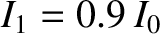be the horizontal displacement of the mass, and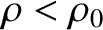the horizontal displacement of the piston. The extension of the spring is thus, assuming that the spring is unstretched when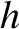. Thus, the horizontal force acting on the mass can be written [cf., Equation (62)]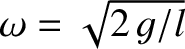(99)

The equation of motion of the system then becomes [cf., Equation (63)](100)

where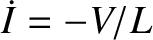is the damping constant, and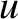the undamped oscillation frequency. Suppose, finally, that the piston executes simple harmonic oscillation of angular frequency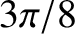and amplitude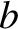, so that the time evolution equation of the system takes the form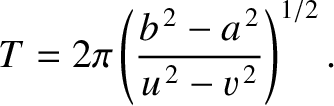(101)

We shall refer to the preceding equation as the driven damped harmonic oscillator equation.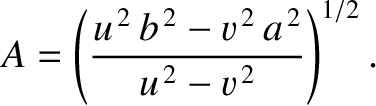We would generally expect the periodically driven oscillator shown in Figure 9 to eventually settle down to a steady (i.e., constant amplitude) pattern of oscillation, with the same frequency as the piston, in which the frictional energy loss per cycle is exactly matched by the work done by the piston per cycle. (See Exercise 9.) This suggests that we should search for a solution to Equation (101) of the form(102)

Here,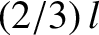is the amplitude of the driven oscillation, whereasis the phase lag of this oscillation (with respect to the phase of the piston oscillation). Because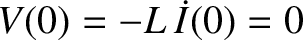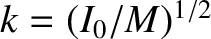(103)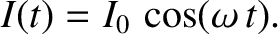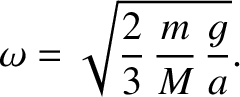(104)

Equation (101) becomes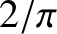(105)

However,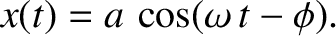and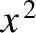(see Appendix B), so we obtain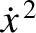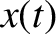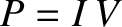(106)

The only way in which the preceding equation can be satisfied at all times is if the (constant) coefficients of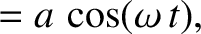and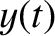separately equate to zero. In other words, if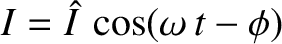(107)(108)

These two expressions can be combined to give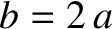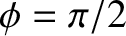(109)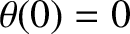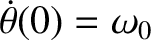(110)

This follows because Equation (108) gives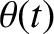(111)

and so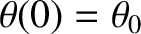(112)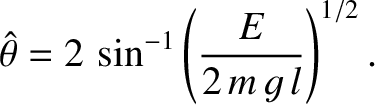(113)

(See Appendix B.) Hence, substitution into Equation (107) gives Equation (109).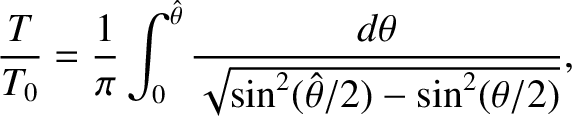Let us investigate the dependence of the amplitude,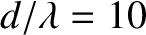, and phase lag,, of the driven oscillation on the driving frequency,. This is most easily done graphically. Figure 10 showsandplotted as functions offor various different values of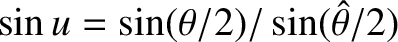. In fact,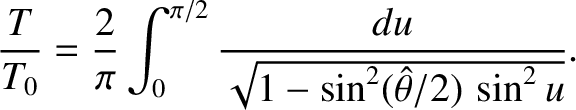,,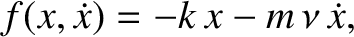,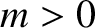, and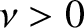correspond to the solid, dotted, short-dashed, long-dashed, and dot-dashed curves, respectively. It can be seen that as the amount of damping in the system is decreased the amplitude of the response becomes progressively more peaked at the system's natural frequency of oscillation,. This effect is known as resonance, andis termed the resonant frequency. Thus, a weakly damped oscillator (i.e.,) can be driven to large amplitude by the application of a relatively small amplitude external driving force that oscillates at a frequency close to the resonant frequency. The response of the oscillator is in phase (i.e.,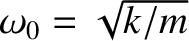) with the external drive for driving frequencies well below the resonant frequency, is in phase quadrature (i.e.,) at the resonant frequency, and is in anti-phase (i.e.,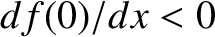) for frequencies well above the resonant frequency.

According to Equations (89) and (109),(114)

In other words, if the driving frequency matches the resonant frequency then the ratio of the amplitude of the driven oscillation to that of the piston oscillation is equal to the quality factor,. Hence,can be interpreted as the resonant amplification factor of the oscillator. Equations (109) and (113) imply that, for a weakly damped oscillator (i.e.,) which is close to resonance [i.e.,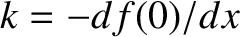],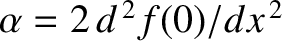(115)

This follows because. Hence, the width of the resonance peak (in angular frequency) is, where the edges of the peak are defined as the points where the driven amplitude is reduced toof its maximum value: that is,. The phase lag at the low and high frequency edges of the peak are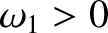and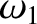, respectively. Furthermore, the fractional width of the peak is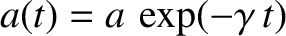(116)

We conclude that the height and width of the resonance peak of a weakly damped () harmonic oscillator scale asand, respectively. Thus, the area under the resonance peak stays approximately constant asvaries.

The force exerted on the system by the piston is(117)

Hence, the instantaneous power absorption from the piston becomes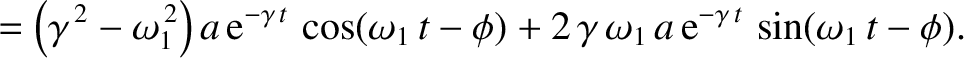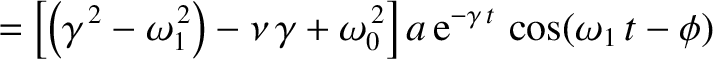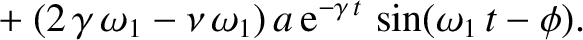(118)

The average power absorption during an oscillation cycle is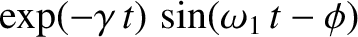(119)

because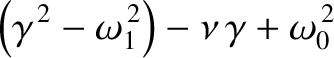and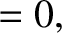. Given that the amplitude of the driven oscillation neither grows nor decays, the average power absorption from the piston during an oscillation cycle must equal the average power dissipation due to friction. (See Exercise 9.) Making use of Equations (114) and (115), the mean power absorption when the driving frequency is close to the resonant frequency is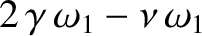(120)

Thus, the maximum power absorption occurs at the resonance (i.e.,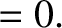), and the absorption is reduced to half of this maximum value at the edges of the resonance (i.e.,). Furthermore, the peak power absorption is proportional to the quality factor,, which means that it is inversely proportional to the damping constant,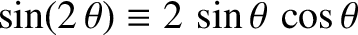.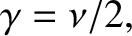Next: Driven LCR Circuits Up: Damped and Driven Harmonic Previous: LCR Circuits
Richard Fitzpatrick 2013-04-08